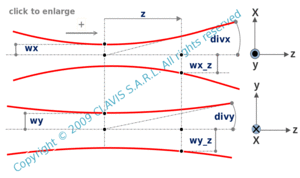and orlaser
elliptical beam

parameters of an elliptical beam ( free )

Caution
Fill in all input cells.
Choose all parameters unit.
Except z, Rh(z) and Rv(z) which are algebric, parameters are strictly positive.
Positive direction is according the horizontal arrow in the bottom right of the scheme.

InputValueunit
wh
wv
z
lam
OutputValueUnit
zohto be calculated
zovto be calculated
wh(z)to be calculated
wv(z)to be calculated
divhto be calculated
divvto be calculated
Rh(z)to be calculated
Rv(z)to be calculatedwh : half width of the waist in the horizontal plane wv : half width of the waist in the vertical plane z : distance from the waist lam : wavelength zoh : Rayleigh range in the horizontal plane zov : Rayleigh range in the vertical plane wh(z) : half width of the beam in the horizontal plane at a distance z from the waist wv(z) : half width of the beam in the horizontal plane at a distance z from the waist divh : half beam divergence in the horizontal plane divv : half beam divergence in the vertical plane Rh(z) : wavefront radius in the horizontal plane Rv(z) : wavefront radius in the vertical plane

Note
This calculation page can not detect all unrealistic inputs.